# Check if the tree has duplicate value or not

In this article, we are going to see whether the tree has a duplicate value or not?
Submitted by Radib Kar, on August 12, 2020

Prerequisite:

To check whether the tree consists of duplicate values or not, the only way is to do any traversal and to store all values in a hash table. While traversing and adding if we find that the value is already present in the hash table, then that means that the tree has duplicate values. If even after the traversal finishes if we don't find any values repeating, then there are no duplicates in the tree.

Below is the two examples, where in the first tree there is a duplicate, but in the second example, there is no duplicate in the tree.

Examples:

1) A binary tree having duplicate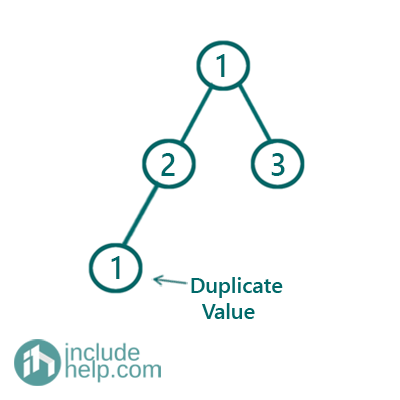Figure 1: Example1 tree

In the above example, we can see that the tree has a duplicate value that is 1.

2) A binary tree without having duplicateFigure 2: Example2 tree

In the above example, we can see that the tree has no duplicate values.

C++ implementation:

```#include <bits/stdc++.h>
using namespace std;

// tree node is defined
class TreeNode {
public:
int val;
TreeNode* left;
TreeNode* right;
TreeNode(int data)
{
val = data;
left = NULL;
right = NULL;
}
};

bool isThereDuplicate(TreeNode* root)
{
if (!root)
return false;

//do level order traversal and add
//the node values to hash table
queue<TreeNode*> q;
q.push(root);
set<int> hash;

while (!q.empty()) {
TreeNode* cur = q.front();
q.pop();
//then duplicate found
if (hash.find(cur->val) != hash.end())
return true;
hash.insert(cur->val);
if (cur->left)
q.push(cur->left);
if (cur->right)
q.push(cur->right);
}

return false;
}

int main()
{
//building the tree like example 1
TreeNode* root1 = new TreeNode(1);
root1->left = new TreeNode(2);
root1->right = new TreeNode(3);
root1->left->left = new TreeNode(1);

if (isThereDuplicate(root1))
cout << "Tree in example 1 has duplicate values\n";
else
cout << "Tree in example 1 doesn't have duplicate values\n";

//building the tree-like example 2
TreeNode* root2 = new TreeNode(1);
root2->left = new TreeNode(2);
root2->right = new TreeNode(3);
root2->left->left = new TreeNode(4);
root2->left->right = new TreeNode(5);
root2->right->right = new TreeNode(6);

if (isThereDuplicate(root2))
cout << "Tree in example 2 has duplicate values\n";
else
cout << "Tree in example 2 doesn't have duplicate values\n";

return 0;
}
```

Output:

```Tree in example 1 has duplicate values
Tree in example 2 doesn't have duplicate values
```

Dry run of examples:

As discussed we will do level order traversal and store the values in the hash table and check for the duplicate. To see detail on hash table and level order traversal, please check the prerequisite articles.

Example 1:

Iteration 1: After processing root 1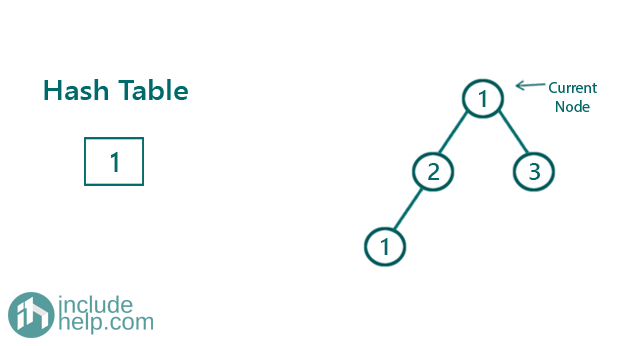Iteration 2: After processing node 2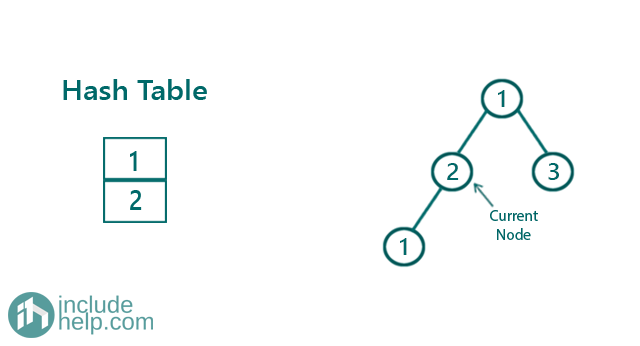Iteration 3: After processing node 3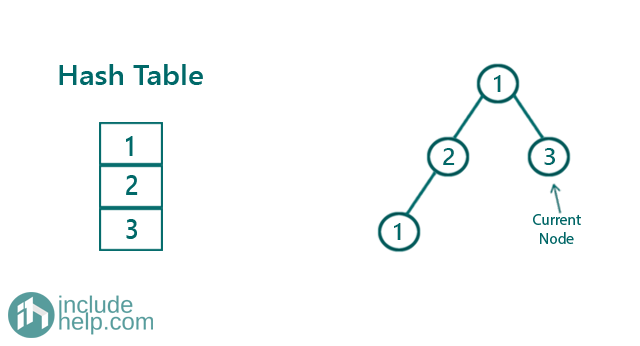Iteration 4: After processing node 1Here we find the duplicate in the tree.

Example 2:

Iteration 1: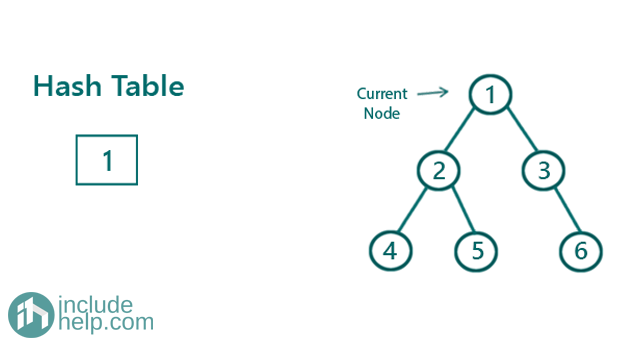Iteration 2: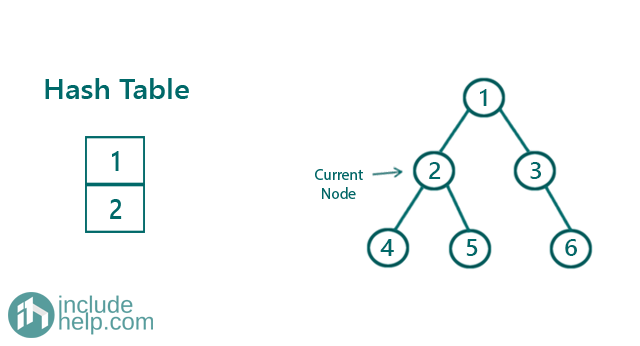Iteration 3: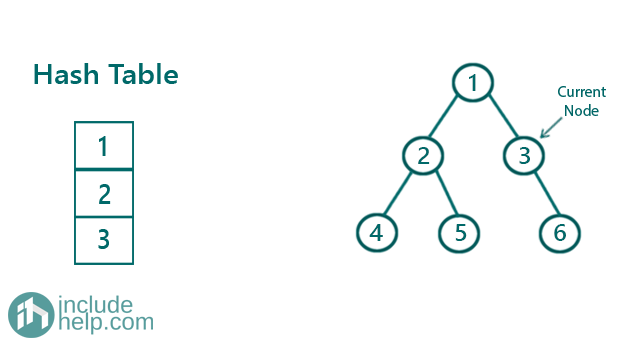Iteration 4: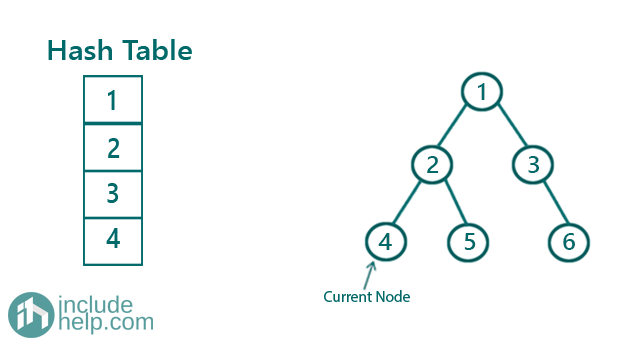Iteration 5: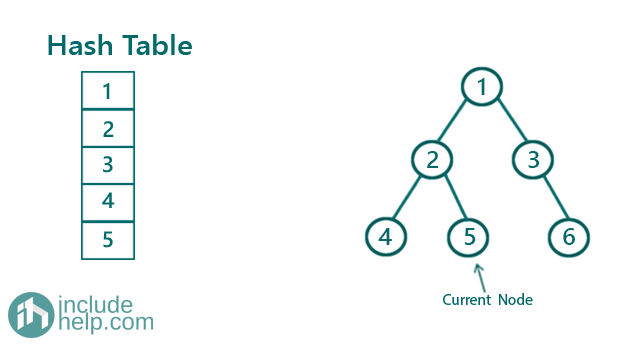Iteration 6: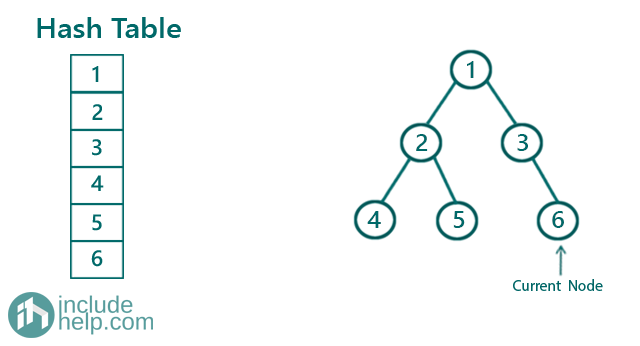So, there is no single duplicate found.